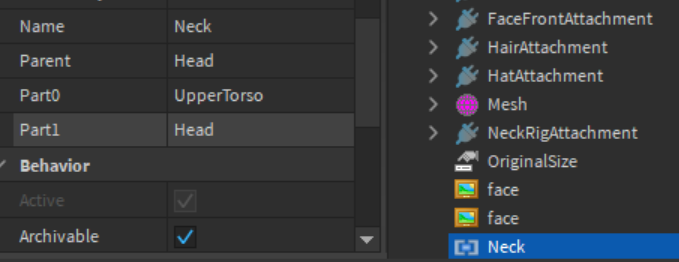# Help on Visualizing a vector?

I can’t understand this specific piece of code.although i wrote.But I can’t understand how this works.I tried searching many times, but can’t understand it.If you guys can help me understand this.It would be grateful.

But what I want to know is How does the piece of code affects the head movement…
as an example take a look at this script…

``````local camDirection = Humanoid_Root_Part.CFrame:toObjectSpace(Camera.CFrame).LookVector

neck.C0 = CFrame.new(0, yOffset, 0) * CFrame.Angles(camDirection.Y, -camDirection.X, 0)

waist.C0 = CFrame.new(0, yOffset2, 0) * CFrame.Angles(camDirection.Y, -camDirection.X, 0)

``````

I know that It sets the root part’s CFrame relative to the camera’s CFrame.But I want to know how this affects the head’s movement. Help me by visualizing this or by explaining.

1 Like

This resource should help visualize a vector, using the .Visualize function:

You can do it by doing this:

``````function visualize(v3, origin, color, thickness, transparency)
local origin = origin or Vector3.new()
local part = Instance.new("Part")
part.Name = "Vector"
part.BrickColor = color or BrickColor.Random()
part.Transparency = transparency or 0
part.Anchored = true
part.Size = Vector3.new(thickness or 0.2, thickness or 0.2, v3.magnitude)
part.CFrame = CFrame.new((origin+v3/2), origin+v3)
part.Locked = true
part.Parent = workspace
return part
end

local camDirection = Humanoid_Root_Part.CFrame:toObjectSpace(Camera.CFrame).LookVector

local visualizerPart = visualize(camDirection, Humanoid_Root_Part.Position)
game.Debris:AddItem(visualizerPart,0.5)--dirty but just for debugging purposes
``````
4 Likes

I tried this.But what I want to know is How does the piece of code affects the head movement…
as an example take a look at this script…

``local camDirection = Humanoid_Root_Part.CFrame:toObjectSpace(Camera.CFrame).LookVector

neck.C0 = CFrame.new(0, yOffset, 0) * CFrame.Angles(camDirection.Y, -camDirection.X, 0)

waist.C0 = CFrame.new(0, yOffset2, 0) * CFrame.Angles(camDirection.Y, -camDirection.X, 0)
``

1 Like

@BuilderBudCarl Can u help me to understand this…?

1 Like

Ok I’ll list out the things the code does, and you’ll point out where you are confused at.

1. First is the object space piece of code which converts the cameras look direction relative to the humanoid root part cframe very similar to the point to object space gif in this article though not exactly.

this is being doing to find the look vector of the camera relative to the humanoid root part:

``````local camDirection = Humanoid_Root_Part.CFrame:toObjectSpace(Camera.CFrame).LookVector

``````

The next tricky part is using C0’s with a Motor6D. This is an entirely different beast that has confused a lot of people like me before.

C0’s of a Motor6D is relative to the Part0. For the neck motor this will be the upper torso and basically we can asume upper torso CFrame will be the same as the root part since it doesn’t tend to move that often.Usually we want to control the Part1 of the Motor6D which is the Head without disconnecting the motor or else the character will die and other bad stuff.

One way to do it is to apply a CFrame to the Motor6D C0. But the issue is the C0 is relative to the Part0. This is why the CFrame:toObjectSpace was done with the humanoid root part.

If you remove it and just use Camera.LookVector it’ll break because it’s relative to object space.

Now the next question is, why these two numbers in * CFrame.Angles(camDirection.Y, -camDirection.X, 0)?

The first one is self explanatory CFrame.new(0, yOffset, 0), puts Head above the torso in the correct place and position.

Now what about the camDirection and what are those two numbers? Personally I haven’t a clue what the original script writers were thinking and it’s getting late for me/

I would start by analyzing the relationship between these numbers and where the camera is looking by printing them out.

`````` CFrame.Angles(camDirection.Y, -camDirection.X, 0)
--replace with
CFrame.Angles(camDirection.Y, 0, 0)--focus one number at a time
print(camDirection.Y)--print it out.
``````

After that start noticing some relationships like CFrame.Angles is noted to accept angles in radians, and this isolated part of the script controls the head up and down movement.

You notice if Cframe.Angles is accepting an angle then what exactly is the angle we are inputting into the CFrame? I prefer using degrees so I will start printing in degrees then experiment again,

``````print(math.deg(camDirection.Y))
``````

You will notice when you point the camera up the angle will be around 50 degrees and when you point the camera down the angle will be around -50 degrees. Therefore I gurss the original script writers intent was to capture this sort of relationship between camera direction and angle, but the exact theory and where the numbers are coming from is still not clear. Tbh, I’m guessing that thus method is just a guesswork with not much mathematical basis to get the angles where the camera is pointing via the direction component of the camera in object space but it gets a guess work of the numbers as it’s not exact compared to the other two post I linked below:

I would continue experimenting with camDirection.X but yeah am tired.

And honestly I don’t like this method, you cannot effectively control the .LookVector of a camera directionI cannot exactly explain why…forgot as normally you expect a measurement method like these two other posts: I would prefer using this angle measurement in this turret example method as I could at least draw out a diagram for it and understand where the angles are coming from, then you can play around with it like dividing it by /1.5 in order to make the head turn less and such so yeah go for this method of getting an angle instead, much more understandable.

But yeah hope this helps? Probably too confusing, it’s intended to capture my train of thought when working on a CFrame problem which is a mess, hopefully someone has a better method and is willing to explain it better good night.

6 Likes

thnx brother.! u saved me.!1 Like